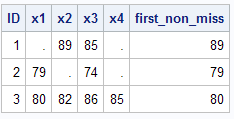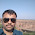# SAS : COALESCE Function

This tutorial explains the usage of COALESCE function and how to use it.

COALESCE

The COALESCE function is used to select the first non-missing value in a list of variables. In other words, it returns the first non-blank value of each row.

Let's create a sample dataset in SAS to understand COALESCE function.

Sample Data
data temp;
input ID x1-x4;
cards;
1 . 89 85 .
2 79 . 74 .
3 80 82 86 85
;
run;
COALESCE : First Non-Missing Value
data want;
set temp;
first_non_miss = coalesce(of x1-x4);
run;
If you look at the output shown in the image below, you would find COALESCE returns 89 in first observation which is the first non-missing value among x1= . , x2=89 , x3=85, x4 = .SAS : COALESCE Function

We can also use COALESCE function in PROC SQL.
proc sql;
select *, coalesce(x1,x2,x3,x4) as first_non_miss
from temp;
quit;

Last Non-Missing Value

Suppose you need to calculate last non-missing value instead of first non-missing value. Unfortunately, there is no such function which returns last non-missing value. To accomplish this task, we can reverse a list of variables and ask SAS to calculate first non-missing value. It would be equivalent to last non-missing value. Indirectly, we are asking SAS to read variables from right to left rather than left to right.
data want;
set temp;
last_non_miss = coalesce(of x4-x1);
run;
Note : coalesce(of x4-x1) is equivalent to coalesce(x4, x3, x2, x1).Last Non-Missing Value
In this case, COALESCE returns 85 as it is a first non-missing value (read from right to left) among x4= . , x3= 85, x2=89, x1= . .Share
Related PostsDeepanshu founded ListenData with a simple objective - Make analytics easy to understand and follow. He has over 10 years of experience in data science. During his tenure, he has worked with global clients in various domains like Banking, Insurance, Private Equity, Telecom and Human Resource.

9 Responses to "SAS : COALESCE Function"
1.can you include more helpful examples

2.Didnt get this

1.3.4.example is great

5.Loved the course so far. Everything is explained in a very simple & detailed way making it easier to understand. One thing I would like to add here is that coalesce can be used to replace missing values with some specific value as well.

1.Yes we can do the same...

6.COALESCE for numeric variables and COALESCEC is for character variables.

1.Next → ← Prev